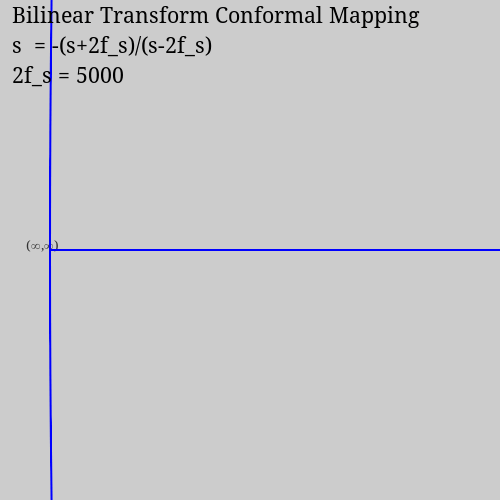# The Geometry of the Bilinear Transform

I'm taking Dan Boschen's DSP for Software Radio class now and it covers the bilinear transform for mapping between the s-plane and z-plane:

$s = \frac{2(z-1)}{T(z+1)}$

The plot for the Z-plane after the mapping looks a lot like a Smith chart, which I posted about previously. Specifically, it resembles an admittance chart, which is a Smith chart rotated 180 degrees. In a Smith chart, the right-hand side of the s-plane gets mapped to the inside of the unit circle. In the bilinear transform, the left-hand side of the s-plane gets mapped to the inside of the unit circle.

I wanted to make another animation which shows how that looks, so I updated my Julia code to do that.

Solving for $$z$$ gives:

$z = -\frac{s+2/T}{s-2/T}$

This conformal mapping is another Mobius transformation $$(a z + b)/(c z + d)$$ with $$a=-b=1$$ and $$d=b=2/T$$.Once again, I had to work out the math for how the vertical and horizontal lines of the Cartesian grid map to the z-plane with this new equation. This time all the vertical lines map to circles with centers on the real axis to the left of (0,0), and they all pass through (-1,0). The horizontal lines map to circles centered above (-1,0).

By chance, the animation is now centered on the z-plane location where s-plane points at infinity map to (the left hand side of the unit circle), not s=(0,0) as in my Smith chart animations. Since I only draw a finite number of grid lines, the left-hand side of the circle at (∞,∞) is rather sparse to begin with, until more and more grid lines near the s-plane come closer as the transform tightens.

I don't have the time to sort that out and recenter the plot on the (0,0) point. But I also want to keep it this way since this new plot also gives a different perspective on the situation.

## The code

Here's the code for this animation:

# Draw a series of Mobius transformations of a regular Cartesian grid
# to illustrate how a bilinear transform conformal map is formed and
# how it relates to Cartesian space.
#

using Graphics, Cairo

plot_width  = 500
plot_height = 500
plot_scale  = 4
clip = true

c = CairoRGBSurface(plot_width, plot_height);
cr = CairoContext(c);

z(s,n) = -(s+n)/(s-n)           # Map complex s to complex z

arc(ctx, xc, yc, radius, 0, 2*pi)

function map_vertical_line(x, n)
set_line_width(cr, x==0 ? 2 : 0.5)
max_diam = 2*n
z_ = z(x+0*im,n)            # z point

z_x = n*real(z_)
z_y = n*imag(z_)

r = (z_x+n)/2
xc = r
yc = 0
circle(cr, xc, yc, r);
stroke(cr)
end

function map_horizontal_line(y, n)
set_line_width(cr, y==0 ? 2 : 0.5)
if (y==0)                   # Special case: x-axis
if (clip)
move_to(cr, 0, 0)
line_to(cr, 2*n, 0)
else
move_to(cr, -plot_width, 0)
line_to(cr,  plot_width, 0)
end

stroke(cr)
return
end

z_ = z(0+y*im,n)
z_x = n*real(z_)
z_y = n*imag(z_)

yc = ((-z_x-n)^2 + z_y^2)/(2*z_y)
xc = 0
r = abs(yc)

if (clip)
arc_deg = abs(2*atan(-z_y, -z_x-n))
if (yc<0)
arc(cr, xc, yc, r, end_deg+arc_deg, end_deg);
else
arc_negative(cr, xc, yc, r, end_deg-arc_deg, end_deg);
end
else
circle(cr, xc, yc, r);
end

stroke(cr)
end

function text_vc_hr(cr, x, y, str)
extents = text_extents(cr, str)
xt = x - (extents + extents)
yt = y + extents/2
text(cr, xt, yt, str)
move_to(cr, 0, 0)           # End the path.
end

function text_vb_hc(cr, x, y, str)
extents = text_extents(cr, str)
@show str, extents
xt = x + (extents/2 - extents)/4
yt = y
text(cr, xt, yt, str)
move_to(cr, 0, 0)           # End the path.
end

function plot_conformal_map(n)
set_source_rgb(cr,0.8,0.8,0.8) # Light gray background.
rectangle(cr,0.0,0.0,plot_width,plot_height)
fill(cr)

# Setup graphics transform matrix, and label points of interest.
save(cr)
translate(cr, (plot_width-400) / 2, plot_width/2) # Center the plot.
set_font_face(cr, "Noto Serif 10")
set_source_rgb(cr, 0.2,0.2,0.2)
text_vc_hr(cr, 0, 0, "(∞,∞)")                     # Label (∞,∞).
scale(cr, plot_scale, plot_scale)                 # Embiggen.
text(cr, n*(1+real(z(50,n))), 0, " (2f_s,0)")     # Label (2f_s,0).
move_to(cr, 0, 0)

# Map and plot the grid lines.
set_source_rgb(cr, 0,0,1)
step = 10
foreach(x -> map_vertical_line(x,n), -50*step:step:0)
foreach(y -> map_horizontal_line(y,n), -50*step:step:50*step)
restore(cr)

# Label plot.
set_source_rgb(cr, 0,0,0)
set_font_face(cr, "Noto Serif 16")
line_space = 30
text(cr, 12, 1*line_space, "Bilinear Transform Conformal Mapping")
text(cr, 12, 2*line_space, "s  = -(s+2f_s)/(s-2f_s)")
text(cr, 12, 3*line_space, "2f_s = $n") # Write it out to a file. write_to_png(c,"bilinear_transform_$n.png")
end

foreach(plot_conformal_map, 50:1:55)
foreach(plot_conformal_map, 55:5:80)
foreach(plot_conformal_map, 80:10:100)
foreach(plot_conformal_map, 200)
foreach(plot_conformal_map, 300:200:1000)
foreach(plot_conformal_map, 1000:1000:5000)